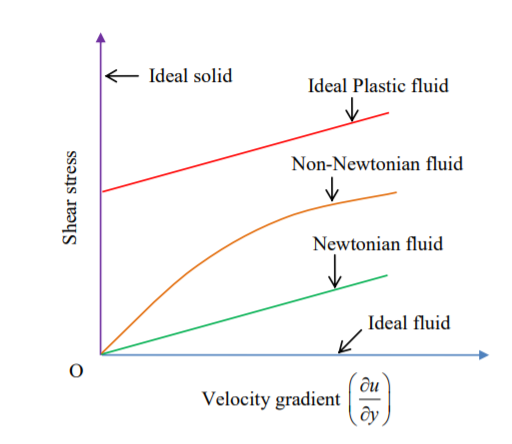# What is Fluid? & types of Fluid.

## What is Fluid?

A Fluid is a substance that continually flows when an external force is applied. Fluids generally include liquids, gases and plasmas. To some extent, plastic solids are also considered fluids.

### Fluids can be classified based on the following properties:

• Viscosity
• Conductivity
• density
• compressible or not.

### Common types of Fluids based on Viscosity:

Fluids are separated in five basic types:

1. Ideal Fluid
2. Real Fluid
3. Newtonian Fluid
4. Non-Newtonian Fluid
5. Ideal Plastic Fluid1. ### Ideal Fluid:

An Ideal Fluid is a fluid which is incompressible in nature and that has no viscosity. In practical, no fluid is ideal fluid because all the fluids have some viscosity. Thus, it is also called as Imaginary Fluid.

1. ### Real Fluid:

Real fluids are the fluids which have some viscosity and are compressible in nature. All the fluids in actual are real fluids.

Examples: Kerosene, Petrol, Castor oil

1. ### Newtonian Fluid:

Newtonian fluids are the fluids that obey Newton’s law of viscosity. In other words, a real fluid whose shear stress is directly proportional to the rate of shear strain is known as Newtonian Fluid. For a Newtonian fluid, viscosity totally depends upon the temperature and pressure of the fluid.

Examples: water, air, emulsions, Hydrogen

Main characteristics:

• Newtonian fluids do not have any elastic properties.
• They are incompressible, isotropic and unreal.
• Viscosity is temperature dependant,
• Viscosity further depends on the various pressures at which it is found.
• At a fixed temperature, their viscosity remains constant.
• With the increase in the temperature of a fluid, the viscosity decreases.
• The viscosity of this type of fluid is inversely proportional to the increase in its temperature.
• The Newtonian fluid was named after Sir Isaac Newton, who defined it as a viscous flow.
• They comply with Newton’s law of viscosity.
1. ### Non-Newtonian Fluid:

Non-Newtonian fluids are the fluids that do not obey Newton’s law of viscosity. In other words, a real fluid in which shear stress is not directly proportional to the rate of shear strain is known as Non-Newtonian Fluid.

Examples: Flubber, Oobleck

#### Non-Newtonian fluids can further be classified as:

1. Time Dependent Fluids: These fluids are the ones for whose shear stress or viscosity decreases with time due to isothermal conditions and steady shear are known as Thixotropic and those that increase with time under same circumstances are known as Rheopectic or Anti-thixotropic.
2. Time Independent Fluids: These fluids are the ones for which the rate of shear at a given point depends on the instantaneous shear stress at that point. These are also known as Non-Newtonian viscous fluids or Purely viscous fluids.

#### These fluids are further of two types:

• Fluids with yield stress
• Fluids without yield stress: These also have two kinds-
• Pseudoplastic Fluids
• Dilatant Fluids

3. Visco-elastic Fluids

### 5. Ideal Plastic Fluid:

Ideal plastic fluid is a fluid, the shear stress is proportional to the rate of shear strain and in which shear stress is more than the yield value, is known as ideal plastic fluid.

Common types of Fluids based on density:

1. Gas
2. Liquid

There are two approaches to study the behaviour of fluid:

1. Lagrangian Approach: In this method, a single fluid particle is taken and studied carefully for its behaviour at varying size of sections.
2. Eulerian Approach: In this approach, fluid is passed through a section of fixed size and then behaviour of fluid particles are studied at different instants.

Visit ourChannel for more information.

Tutorials tips

### 4 Responses

1.Louis Christopher says:

Good work

2.Samuel says:

Nice one

1. July 22, 2021

[…] shear stress is not proportional to the rate of velocity gradient (shear strain) is known as a Non-Newtonian fluid. It does not obey newton’s law of […]

2. July 24, 2021

[…] shear stress is not proportional to the rate of velocity gradient (shear strain) is known as a Non-Newtonian fluid. It does not obey newton’s law of […]